# Bihar Board Class 12 Chemistry Solutions Chapter 6 General Principles and Processes of Isolation of Elements

Bihar Board Class 12 Chemistry Solutions Chapter 6 General Principles and Processes of Isolation of Elements Textbook Questions and Answers, Additional Important Questions, Notes.

## BSEB Bihar Board Class 12 Chemistry Solutions Chapter 6 General Principles and Processes of Isolation of Elements

### Bihar Board Class 12 Chemistry General Principles and Processes of Isolation of Elements Intext Questions and Answers

Question 1.
Which of the ores mentioned in Table 6.1 could be concentrated by magnetic separation method?
Ores in which one of the components (either the impurity or the actual ore) is magnetic can be concentrated, e.g, ores containing iron (hematite, magnetite, siderite and iron pyrites).

Question 2.
What is the significance of leaching in the extraction of aluminium?
Leaching is significant in the extraction of aluminium from the bauxite ore as it is usually contaminated with impurities like SiO2, Fe2O3 etc. The powdered ore is digested with a concentrated solution of NaOH at 473-523 K and 35-36 bar pressure.
Al2O3 (S) + 2 NaOH (aq) + 3H2O (l) → 2 Na [Al (OH)4] (aq).

SiO2 is also leached out as sodium silicate, leaving other impurities like Fe2O3 behind. The aluminate in solution is neutralised by CO2 gas and hydrated Al2O3 is precipitated, whereas sodium silicate remains in the solution
2 Na [ Al (OH)4 (aq) + CO2 (g)] → Al2O3 x H2O + 2 NaHCO3 (aq).
Hydrated alumina Al2O3 x H2O is filtered, dried and heated to give back pure Al2O3.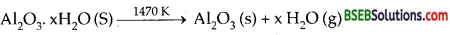Question 3.
The reaction Cr2O3 + 2Al → Al2O3 + 2Cr [ΔG° = – 421 KJ] is thermodynamically feasible as is apparent from the Gibbs energy value. Why does it not take place at room temperature?
Certain amount of activation energy is essential even for such reactions which are thermodynamically feasible. Therefore for the reaction heating is required.
Cr2O3 + 2Al → Al2O3 + 2Cr

Question 4.
Is it true that under certain conditions, Mg can reduce SiO2 and Si can reduce MgO? What are those conditions?
Yes, below 1773 K, Mg can reduce SiO2 Si can reduce MgO above 1773 K. This can be inferred from the graph of ΔG° Vs T [Ellingham diagram].### Bihar Board Class 12 Chemistry General Principles and Processes of Isolation of Elements Text Book Questions and Answers

Question 1.
Copper can be extracted by hydrometallurgy but not zinc. Explain.
Copper can be extracted by hydrometallurgy. The solution is leached out using acid. The solution containing Cu2+ is treated with H2.
Cu2+ (aq) + H2 (g) → Cu (S) + 2H+ (aq)
Zinc cannot be extracted by hydrometallurgy as zinc is highly reactive metal. It may not be possible to replace it from a solution.

Question 2.
What is the role of depressant in froth floatation process?
If a mixture of two sulphides (say, ZnS and PbS) are to be separated and concentrated by Froth Floatation process, a substance called depressant (in this case NaCN) is used. It selectively prevents ZnS from coming to the froth but allows PbS to come up with the froth.

Question 3.
Why is the extraction of copper from pyrites more difficult than that from its oxide ore through reduction?
The Gibbs energies of formation of most sulphides are greater than for Cu2O. In fact Cu2O is an endothermic compound. Hence it is a common practice to roast sulphide ores to corresponding oxides prior to reduction.

Question 4.
Explain (1) Zone-refining, (2) Column Chromatography.
1. Zone Refining-This method is based on the principle that the impurities are more soluble in the melt than in the solid-state of the metal. A circular mobile heater is fixed at one end of a rod of the impure metal (Fig.). The molten zone moves along with the heater which is moved forward. As the heater moves forward, the pure metal crystallises out of the melt and the impurities pass on into the adjacent molten zone. The process is repeated several times and the heater is moved in the same direction. At one end, impurities get concentrated. This end is cut off. This method is very useful for producing semiconductor and other metals of very high purity, e.g., germanium, silicon, boron, gallium and indium.2. Column Chromatography-This method is based on the principle that different components of a mixture are differently adsorbed on an adsorbent. The mixture is put in a liquid or gaseous medium which is moved through the adsorbent. Different components are adsorbed at different levels on the column. Later the adsorbed components are removed (eluted) by using suitable solvents (eluent). Depending upon the physical state of the moving medium and the adsorbent material and also on the process of passage of the moving medium, the chromatographic method is given the name. In one such method, the column of Al2O3 is prepared in glass tube and the moving medium containing solution of the components is in liquid form.

This is an example of column chromatography. This is very useful for purification of the elements which are available in minute quantities and the impurities are not very different in chemical properties from the element to be purified. There are several chromatographic techniques such as paper chromatography, column chromatography, gas chromatography, etc. Procedures followed in column chromatography have been depicted in Fig.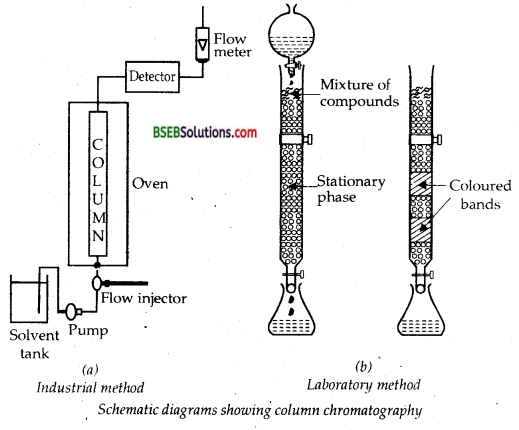Question 5.
Out of C and CO, which is a better reducing agent of 673 K?
Carbon monoxide (CO).

Question 6.
Name the common elements present in the anode mud in electrolytic refining of copper. Why are they so present?
Selenium, tellurium, silver and gold are the metals present in the anode mud below the anode. This is because these are less reactive than copper.Question 7.
Write down the reactions taking place in different zones in the blast furnace during the extraction of iron.
Near the layers, coke and limestone are heated when C bums to give CO2.
C(s) + O2 (g) → CO2 (g); ΔH = – 393.3 kj mol-1
Since the reaction is exothermic, lot of heat is produced and the temperature here is 1673 K.
As the gases move up, they meet the descending charge. The coke present in the charge reduces CO2 to CO.
CO2 + C → 2 CO ↑; ΔH+ = + 163.2 kj
Since the reaction is endothermic, therefore, the temperature falls gradually to about 1423.
Below 1123 K, CO reduces the ores to FeO.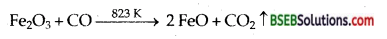Reduction of FeO to Fe by CO occurs at about 1123 K.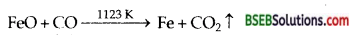In this region lime stone also decomposes to form CaO and CO2.
CaCO3 (s) → CaO (s) + CO2 (g)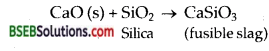Question 8.
Write chemical reactions taking place in the extraction of zinc from zinc blende.
(i) During roasting zinc blends [ZnS] combines with 02 to form ZnO.
2 ZnS + 3O2 → 2 ZnO + 2 SO2

(ii) The reduction of zinc oxide to zinc is done using coke. To raise the temperature to 673 K, the oxide is made into briquettes with coke and day.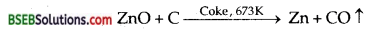The metal is distilled off and collected by rapid chilling.

Question 9.
State the role of silica in the metallurgy of copper.
Silica acts as a flux to remove the impurities [oxides of iron] remaining in the matter by forming silicate, FeSiO3 which is a fusible slag.Question 10.
What is meant by the term “chromatography?”
Chromatography-It is defined as a technique of separating the components of a mixture in which separation is achieved by the differential movement of individual components through a stationary phase under the influence of a mobile phase.

Question 11.
What criterion is followed for the selection of the stationary phase in chromatography?
Different components to be separated from the given mixture should be able to get differently adsorbed on the adsorbent like silica or aluminium gel (SiO2 or Al2O3). The stationary phase should be immobile and immiscible.

Question 12.
Describe a method for refining nickel.
Nickel is refined by MOND process. When impure nickel is heated in a current of CO at 330-350 K it forms volatile nickel carbonyl leaving behind the impurities. The nickel carbonyl [Ni(CO)4] thus obtained is then heated to a higher temperature (450-470 K) when it undergoes thermal decomposition giving pure metal.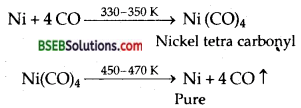Question 13.
How can you separate alumina from silica in a bauxite ore associated with silica? Give equations, if any.
The bauxite ore of aluminium usually contains silica (SiO2) as one of the main impurity. Concentration is carried out by digesting the powdered ore with a concentrated solution of NaOH at 473 – 523 K and 35 – 36 bar pressure. This way, alumina (Al203) is leached out as sodium aluminate (and Si02 too as sodium silicate) leaving the impurities behind.
Al2O3 (s) + 2NaOH (aq) + 3H2O (l) → 2 Na [ Al(OH)4]aq.

The aluminate in solution is neutralised by passing CO2 gas and hydrated Al2O3 is precipitated. At this stage, the solution is seeded with freshly prepared samples of hydrated Al2O3 which induces the precipitation.
2Na [Al (OH)4] (aq) + CO2 (g) → Al2O3. xH2O (s) + 2NaHCO3 (aq)
The sodium silicate remains in the solution and hydrated alumina is filtered, dried and heated to give back pure Al2O3.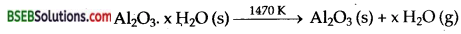Question 14.
Giving examples, differentiate between roasting and calcination.
Roasting-It is the process of converting an ore into its metallic oxide by heating strongly at temperature insufficient to melt in excess of air. The following changes occur:
(i) Moisture from ore is removed.
(ii) Organic matter is destroyed.
(iii) Non-metallic impurities like that of S, P, As are oxidized and are removed as volatile oxides.
(iv) Ores are generally converted into metallic oxides.CALCINATION-It is the process of converting an ore into its oxide by heating it strongly below its melting point either in the absence of air or limited supply of air. The following changes occur:
(i) Moisture is driven out
(ii) Volatile impurities of S, AS, P are removed as their volatile oxides
(iii) Water is removed from hydrated oxides and hydroxide ores.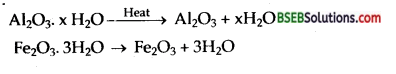where ΔH is the enthalpy change during the reaction, T is the absolute temperature and ΔS is the change in entropy.
Consider the Ellingham diagram (given below) for some metal oxides. From the diagram, it is evident that metals for which the free energy of formation of their oxides is more negative can reduce those metal oxides for which the free energy of formation of their respective oxides is less negative. In other words, any metal will reduce the oxide of other metals which lie above it in the Ellingham diagram because the free energy will become more negative by an amount equal to the difference in the two graphs at that particular temperature. Thus A1 reduces FeO, Cr2O3 and NiO in Thermite reaction, but A1 will not reduce MgO at a temperature below 1773 K.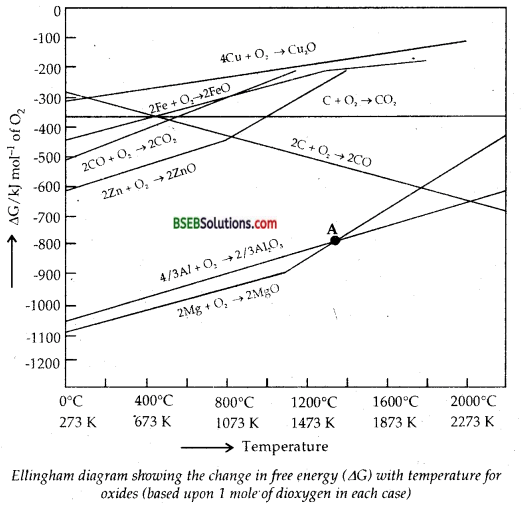It can be followed that: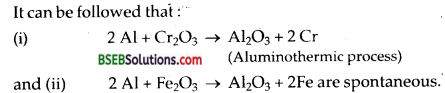But Al can’t be used to reduce MgO below 1500°C. From the above it is clear that thermodynamic considerations help us in choosing a suitable reducing agent in metallurgy.Question 24.
Name the processes from which chlorine is obtained as a by-product. What will happen if an aqueous solution of NaCl is subjected to electrolysis?
(i) Cl2 gas can be obtained in the manufacture of sodium metal from molten sodium chloride wherein it is collected at anode in
Down’s cell.
NaCl (l) → Na+ (l) + Cl (l)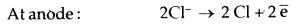2 Cl → Cl2 (g) ↑
At cathode: 2 Na+ + 2e → 2 Na (l)

(ii) It can also be obtained as one of the by-products from the electrolysis of aqueous NaCl (brine solution)
2 Cl (aq) + 2H2O (l) → 2 OH(aq) + H2 (g) + Cl2 (g)
The ΔG° for this reaction is + 422 kj.
when it is converted to E° using ΔG° = – nFE°, we get E° = – 2.2 V.
∴ It will require an external e.m.f. that is > 2.2 V.
But the electrolysis requires an excess potential to overcome some other hindering reactions. Thus Cl2 is obtained along with H2 as a by-product during electrolysis of brine solution.

(b) (i) NaCl(aq) → Na+ (aq) + Cl (aq)
(ii) H2O(l) → H+ (aq) + OH (aq)
At cathode-Both H+ ions and Na+ ions migrate to the cathode. But H+ ions get discharged due to lower deposit potential than Na+.
2H+ + 2e → H2 (g).
At anode-Both OH ions and Cl ions migrate to the anode. The discharge potential of CT ions is lower than that of OH.
∴ CF ions get discharged at anode.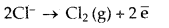Na+ ions and OH combine to form NaOH.

Question 25.
What is the role of graphite rod in the electrometallurgy of aluminium? ,
In the electrometallurgy of Al from Al2O3 (purified and mixed with Na3AlF6 or CaF2) graphite rod is used as anode. The graphite anode is used to reduce Al2O3 to the metal. The overall reaction is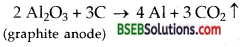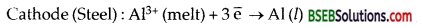Anode (graphite): C(s) + O2- (melt) → CO (g) + 2e
C (s) + 2O2- (melt) → CO2 (g)’+ 4e
For each kg of Aluminium produced 0.5 kg of graphite, anode is burnt away.

Question 26.
Outline the principle of refining of metals by the following methods : (i) Zone-refining, (ii) Electrolytic refining, (iii) Vapour phase refining.
(i) Zone-refining-This method is based on the principle that d the impurities are more soluble in the melt than in the solid-state of the metal. A circular mobile heater is fixed at one end of a rod of the impure metal (Fig.). The molten zone moves along with the heater which is moved forward. As the heater moves forward, the pure metal crystallises out of the melt and the impurities pass on into the adjacent molten zone. The process is repeated several times and the heater is moved in the same direction. At one end, impurities get concentrated. This end is cut off. This method is very useful for producing semiconductor and other metals of very high purity, e.g., germanium, silicon, boron, gallium and indium.(ii) Electrolytic refining In this method, the impure metal is made to act as anode. A strip of the same metal in pure form is used as cathode. They are put in a suitable electrolytic bath containing soluble salt of the same metal. The more basic metal remains in the solution and the less basic ones go to the anode mud. This process is also explained using the concept of electrode potential, overpotential, and Gibbs energy which you have seen in previous sections. The reactions are :
Anode: M → Mn+ + ne
Cathode: Mn+ + ne → M

Copper is refined using an electrolytic method. Anodes are of impure copper and pure copper strips are taken as cathode. The electrolyte is acidified solution of copper sulphate and the net result of electrolysis is the transfer of copper in pure form from the anode to the cathode:
Anode: Cu → Cu2+ + 2 e
Cathode : Cu2+ + 2e → Cu

Impurities from the blister copper deposit as anode mud which contains antimony, selenium, tellurium, silver, gold and platinum; recovery of these elements may meet the cost of refining.
Zinc may also be refined this way.

(iii) Vapour phase refining In this method, the metal is converted into its volatile compound and collected elsewhere. It is then decomposed to give pure metal. So, the two requirements are:
(i) the metal should form a volatile compound with an available reagent, and
(ii) the volatile compound should be easily decomposable so that the recovery is easy.

Following examples will illustrate this technique.
Mond Process for Refining Nickel: In this process, nickel is heated in a stream of carbon monoxide forming a volatile complex, nickel tetracarbonyl: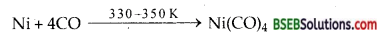The carbonyl is subjected to higher temperature so that it is decomposed giving the pure metal:van Arkel Method for Refining Zirconium or Titanium This method is very useful for removing all the oxygen and nitrogen
present in the form of impurity in certain metals like Zr and Ti. The crude metal is heated in an evacuated vessel with iodine. The metal iodide being more covalent, volatilises:
Zr + 2I2→ Zrl4
The metal iodide is decomposed on a tungsten filament, heated to about 1800K when pure metal is deposited on the filament.
ZrI4 → Zr + 2I2.Question 28.
Predict conditions under which Al might be expected to reduce MgO.
As is clear from Ellingham diagram, Al is expected to reduce MgO only above 1700 K.

### Bihar Board Class 12 Chemistry General Principles and Processes of Isolation of Elements Additional Important Questions and Answers

Question 1.
What is gangue? ‘
The earthy and silicious impurities associated with the ores is called gangue.

Question 2.
What type of ores are roasted?
Sulphide ores.

Question 3.
Why do some metals occur in the native state?
Metals like Au, Pt which are not reactive, i.e., are not attracted by O2, moisture and CO2 of air occur in the native state.

Question 4.
What is a slag?
Gangue combines with flux to form slag.
Gangue + Flux → Slag.

Question 5.
What are Fluxes? How are they useful?
Flux is a substance (acidic or basic) which combines with gangue (basic or acidic respectively) still left in the roasted or calcined ore to form fusible slag.
Flux + gangue → Slag.

Question 6.
What do you mean by beneficiation process?
The process used to concentrate an ore is called beneficiation process.

Question 7.
Which is a better reducing agent at 983 K, carbon or carbon monoxide?
CO. Above 983 K, CO being more stable does not act as a good reducing agent, but carbon does.

Question 8.
Is it true that under certain conditions Mg can reduce SiO2 and Si can reduce MgO?
Below 1773 K, Mg can reduce SiO2 but above 1773 K, Silicon (Si) can reduce MgO.

Question 9.
Write the name of one important ore each of iron and aluminium. Give their formulae.
(i) Iron: Haematite (Fe2O3)
(ii) Aluminium : Bauxite (Al2O3.2H2O).

Question 10.
What is a depressant. Give one example.
Compounds which prevent the formation of froth in froth floatation process are called depressants. For example NaCN.

Question 11.
What is the role of a stabilizer in froth flotation process?
Chemical compounds like cresols and aniline which tend to stabilize the froth are called froth stabilizers.

Question 12.
Indicate the temperature at which carbon can be used to reduce FeO.
Above 1123 K, carbon can be used as a reducing agent for FeO. . . .Question 13.
Define Thermite process/aluminium them.
The reduction of metal oxides likeCr2O3, Fe2O, to their metals with evolution of large amount of heat is called Thermite process/alumino therapy.

Question 14.
What is van Arkel method of obtaining ultra-pure metals?
The impure metal (Ti, Zr) is first converted into its unstable volatile iodide at 523 K which on decomposition at 1700 K gives ultra-pure metal.

Question 15.
What is the principle of zone refining?
When the molten solution of the impure metal is allowed to cool, limit. pure metal crystallises out while the impurities remain in solution.

Question 16.
Write the formula of copper pyrites.
CuFeS2.Question 17.
How can impure copper after extraction is refined?
It is refined electrolytically wherein impure copper is made the anode and the strip of pure copper is made the cathode. The bath is an acidified solution of copper sulphate (CuSO4).

Question 18.
Give examples of an acidic and a basic flux. Give their roles.
Acidic flux-SiO2. It is used to remove basic impurities present in the ore.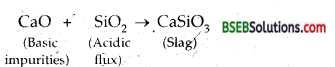Basic flux-limestone (CaCO3). It is used to remove acidic impurities present in the ore.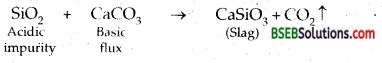Question 19.
Define electrometallurgy.
The process of extraction of metals by electrolysis of their fused salts is called electrometallurgy. Electrons serve as the reducing agent.

Question 20.
Give the equation which can be used to study the thermodynamics of the reactions used in metallurgical operations.
ΔG = ΔH – TΔS,’ where AG is called Gibbs function or Gibbs energy change, ΔH is the enthalpy change, ΔS is the entropy occurring at the temperature T.

Question 1.
Why is it advantageous to roast a sulphide ore before reduction?
The standard free energies (ΔG°) of formation of most of the sulphides are greater than those of CS2 and H2S (CS2 is, in fact, an endothermic compound. Therefore, neither C nor H2 can reduce sulphides to their oxides. In contrast, the standard free energies of formation of oxides are much lower than those of SO2 and hence oxidation of metal sulphides to metal oxides is thermodynamically favourable.

Question 2.
How does NaCN act as a depressant in preventing ZnS from forming the froth?
NaCN combines with ZnS to form a complex Na2 [Zn (CN)4] on the surface of ZnS and thus prevents it from formation of froth.
ZnS + 4 NaCN → Na2 [Zn (CN)4] + Na2S. Sodium tetracyanozincate (II)

Question 3.
What is the principle of Froth floatation process and what type of ores are concentrated by this process?
Froth floatation process is widely used for the concentration of sulphide ores, i.e., Zinc blende (ZnS), Copper pyrites (CuFeS2), galena (PbS) etc. It is based upon the principle of the preferential wetting of ore particles by oil (pine oil) and gangue particles by water. Lighter ore particles form froth and come to the surface whereas gangue particles settle down at the bottom.

Question 4.
What is refining and how liquation process is used for refining of metals?
The process of purifying the crude metals is called refining liquation. Metals like Sn and Pb are purified by this process since the melting points of these metals are lower than those of the impurities.

Question 5.
What is vapour phase refining. Give the reactions involved in the refining of a metal by it?
Vapour phase refining In this method, the impure metal is heated with a suitable reagent at some lower temperature to form a volatile compound leaving behind the impurities. The volatile compound is then heated at a higher temperature when it undergoes decomposition to give the pure metal. For example:
Nickel (Ni) is purified by MOND’s Process.Question 6.
What is Hydrometallurgy? Name metals extracted by it.
Hydrometallurgy is the process of dissolving the ore in a suitable chemical reagent followed by extraction of the metal either by electrolysis or precipitation of the metal by a more electropositive metal. Ag and Au are extracted by this process.
4 Ag + 8 NaCN + O2 + 2H2O → 4 Na [Ag (CN)2]+ 4 NaOH
2 Na [Ag(CN)2] + Zn → Na2 [Zn (CN)4] + 2Ag ↓Question 7.
Which of the following metals cannot be extracted by the smelting process and why? Al, Zn, Fe, Pb. (W. Bengal J.E.E. 2003)
Aluminium (Al) cannot be extracted by the smelting process because of the following reasons:—
(i) Al is a highly electropositive element having strong affinity for oxygen. Therefore Al2O3 is a very stable compound and hence cannot be reduced by carbon.
(ii) Al2O3 when heated with carbon forms aluminium carbiue.
2 Al2O3 + 9 C → Al4C3 + 6 CO ↑

Question 8.
Define Leaching. Which ore is concentrated by leaching.
Leaching is a process by which a particular ore can be dissolved selectively by using certain acids, bases or other reagents.
Bauxite ore [Al2O3] is concentrated by leaching by removing the iron impurities [Fe2O3] and silican present in the bauxite ore.

Question 9.
Cinnabar (HgS) and galena (PbS) on roasting often given their respective metals, but zinc blende (ZnS) does not. Explain.
On roasting all these sulphides are partly converted into their respective oxides. Since the oxides of mercury and lead are unstable while that of zinc is stable therefore, oxides of mercury and lead bring about the reduction of their respective sulphides to the corresponding metals, but ZnO does not reduce ZnS to Zn metal.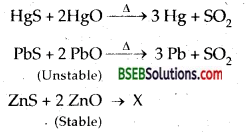Question 10.
What is the major difference between roasting and calcination?
Roasting is the process of heating up an ore in excess of air whereas calcination is the process of heating up of an ore in absence or limited supply of air. In both heating is done below the melting point of the ore.

Question 1.
(a) Explain how an element can be extracted using an oxidation reaction?
(b) What do you mean by refining? Mention some of the methods used for refining of metals.
(a) Some of the extractions, particularly of non-meals are based upon oxidation.
A very common example of extraction based on oxidation is the extraction of chlorine from brine (Chlorine is abundant in seawater as common salt).
2Cl(aq) + 2H2O(l) → 2OH (aq) + H2(g) + Cl2(g)
The ΔG° for this reaction is + 422 kj. When it is converted to E° (using ΔG° = – nE°F), we get E° = – 2.2 V. Naturally, it will require an external e.m.f. that is greater than 2.2 V. But the electrolysis requires an excess potential to overcome some other hindering reactions. Thus, Cl2 is obtained by electrolysis giving out H2 and aqueous NaOH as by-products. Electrolysis of molten NaCl is also carried out.

But in that case, Na metal is produced and not NaOH. The extraction of gold and silver involves leaching the metal with. CN. This is also an oxidation reaction (Ag → Ag+ or Au→ Au+). The metal is later recovered by displacement method.
4Au(s) + 8CN(aq) + 2H2O(aq) + O2(g)→ 4[Au(CN)2] (aq) + 4OH(aq)
2[Au(CN)2] (aq) + Zn(s) → 2Au(s) + [Zn(CN)4]2- (aq)
In this reaction zinc acts as a reducing agent.

(b) A metal extracted by any method is usually contaminated with some impurity. For obtaining metals of high purity, several techniques are used depending upon the difference in properties of the metal and the impurity.
The process is called Refining. Some of them are listed below:

• Distillation,
• Liquation,
• Electrolysis,
• Zone-refining,
• Vapour phase refining,
• Chromatographic methods.

Question 2.
(a) How is concentrated ore converted into metallic form. Give examples.
(b) What are the thermodynamic principles on which metallurgy is based?
(a) The concentrated ore must be converted into a form which is suitable for reduction. Usually, the sulphide ore is converted to oxide before reduction. Oxides are easier to reduce. Thus isolation of metals from concentrated ore involves two major steps viz.,
(a) conversion to oxide, and
(b) reduction of the oxide to metal.
(a)Conversion to Oxide The concentrated ore is either a hydrated oxide, a carbonate or a sulphide. The ore is subjected to calcination or roasting/ smelting to get the oxide.
(i) Calcination-Calcinaton involves heating when the volatile matter escapes leaving behind the metal oxide:(ii) Roasting-In roasting, the ore is heated in a regular supply of air in a furnace at a temperature below the melting point of the metal. Some of the reactions involving sulphide ores are ;
2ZnS + 3O2 → 2ZnO + 2SO2
2PhS +3O2 → 2PbO + 2SO2
2Cu2S + 3O2 → 2CU2O + 2SO2

The sulphide ores of copper are heated in a reverberatory furnace. If the ore contains iron, it is mixed with silica before heating. Iron oxide ‘slags of’ as iron silicate and copper is produced in form of copper matte which contains Cu2S and FeS.
FeO + SiO2 → FeSiO3 (slag)
The SO2 produced is utilised for manufacturing H2SO4.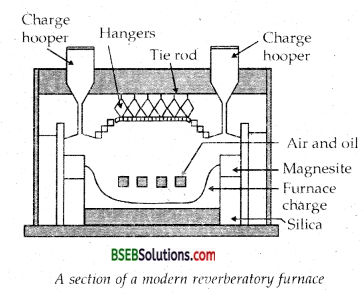(b) Reduction of Oxide to the Metal-Reduction of the metal oxide usually involves heating it with some other substance acting as a reducing agent (C or CO or even another metal). The reducing agent (e.g., carbon) combines with the oxygen of the metal oxide.
MxOy + yC → xM + yCO
Some metal oxides get reduced easily while others are very difficult to be reduced (reduction means electron gain or electronation). In any case, heating is required. To understand the variation in the temperature requirement for thermal reductions (pyrometallurgy) and to predict which element will suit as the reducing agent for a given metal oxide (MxOy), Gibbs energy interpretations are made.

(b) Thermodynamic Principles of Metallurgy-Some basic concepts of thermodynamics help us in understanding the theory of metallurgical transformations. Gibbs energy is the most significant term here. The change in Gibbs energy, AG for any process at any specified temperature, is described by the equation:
ΔG = ΔH – TΔS ……………………………………………….. (1)
where, ΔH is the enthalpy change and AS is the entropy change for the process. For any reaction, this change could also be explained through the equation:
ΔG° = – RTlnK

where, K is the equilibrium constant of the ‘reactant – product’ system at the temperature, T. A negative ΔG implies a +ve K in equation (1). And this can happen only when reaction proceeds towards products From these facts we can make the following conclusions:
1.When the value of ΔG is negative in’ equation (1) then only the reaction will proceed. If ΔS is positive, on increasing the temperature (T), the value of TΔS would increase (ΔH < TΔS) and then AG will become -ve.

2. If reactants and products of two reactions are put together in a system and the net ΔG of the two possible reactions is – ve, the overall reaction will occur. So the process of interpretation involves coupling of the two reactions, getting the sum of their ΔG and looking for its magnitude and sign. Such coupling is easily understood through Gibbs energy (ΔGV) vs T plots for formation of the oxides.Question 3.
How  Ellingham diagram help to explain the extraction of copper from Cuprous oxide [Copper (I) Oxide]?Extraction of Copper from Cuprous oxide [Copper (1) Oxide]:
In the graph of ΔrG° vs T for formation of oxides (Fig.), the Cu2O line is almost at the top. So it is quite easy to reduce oxide ores of copper directly to the metal by heating with coke (both the lines of C, CO and C, C02 are at much lower positions in the graph particularly after 500 – 600K). However most of the ores are sulphide and some way also contain iron . The sulphide ores are roasted / smelted to give oxides:
2Cu2S + 3O2 → 2CU2O + 2SO2
The oxide can then be easily reduced to metallic copper using
coke:
Cu2O + C → 2Cu+CO

In actual process, the ore is heated in a reverberatory furnace after mixing with silica. In the furnace, iron oxide ‘slags of’ as iron silicate and copper is produced in forms of copper matte. This contains Cu2S and FeS. ,
FeO + SiO2 → FeSiO3 (Slag)

Copper matte is then charged into silica lined convertor. Some silica is also added and hot air blast is blown to convert the remaining FeS2, FeO and Cu2S/Cu2O to the metallic copper. Following reactions take place:
2FeS + 3O2 →2FeO + 2SO2
FeO + SiO2 → FeSiO3
2Cu2S + 3O2 → 2Cu2O + 2SO2
2Cu2O + Cu2S → 6Cu + SO2
The solidified copper obtained has blistered appearance due to the evolution of SO2 and so it is called blister copper.